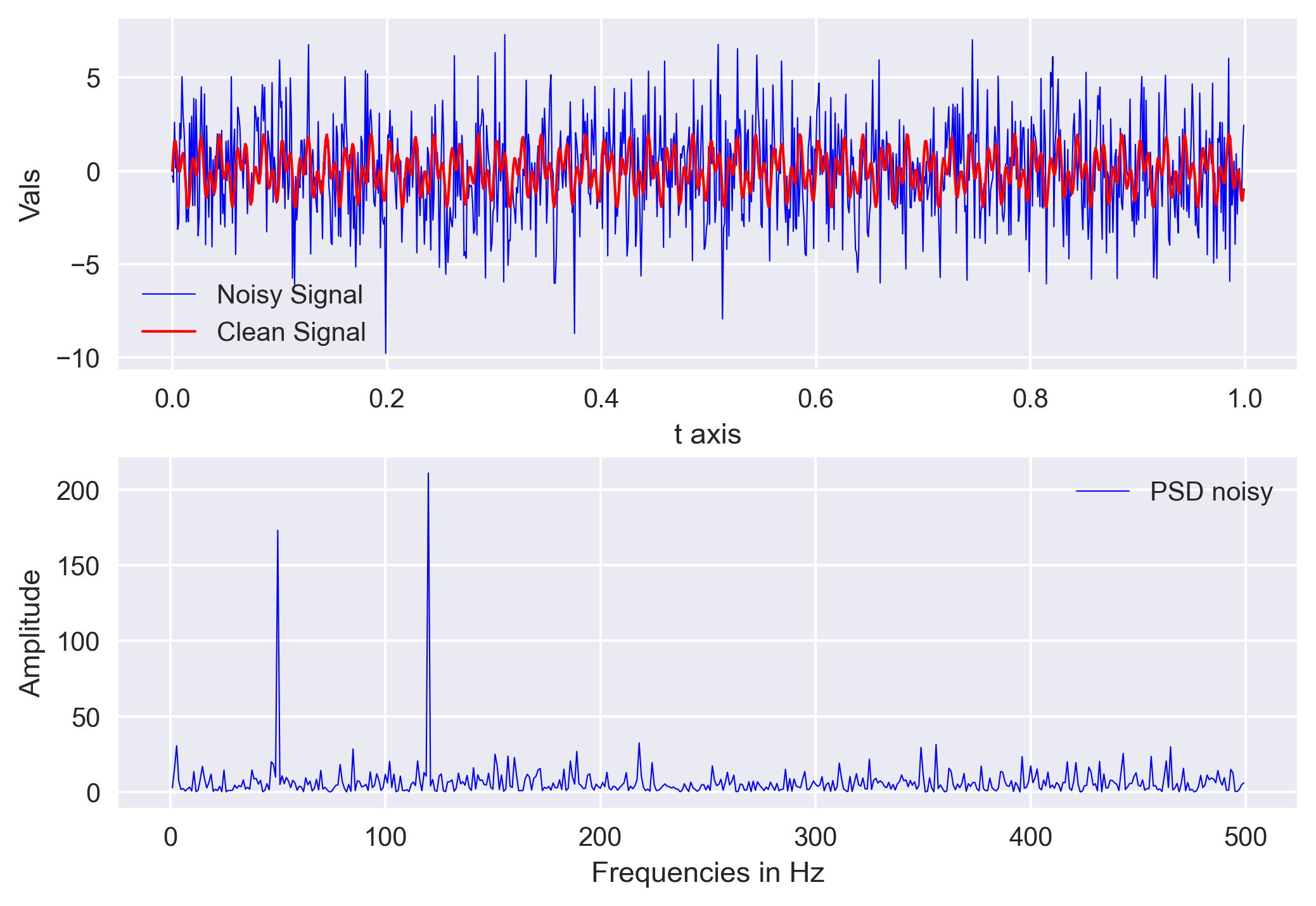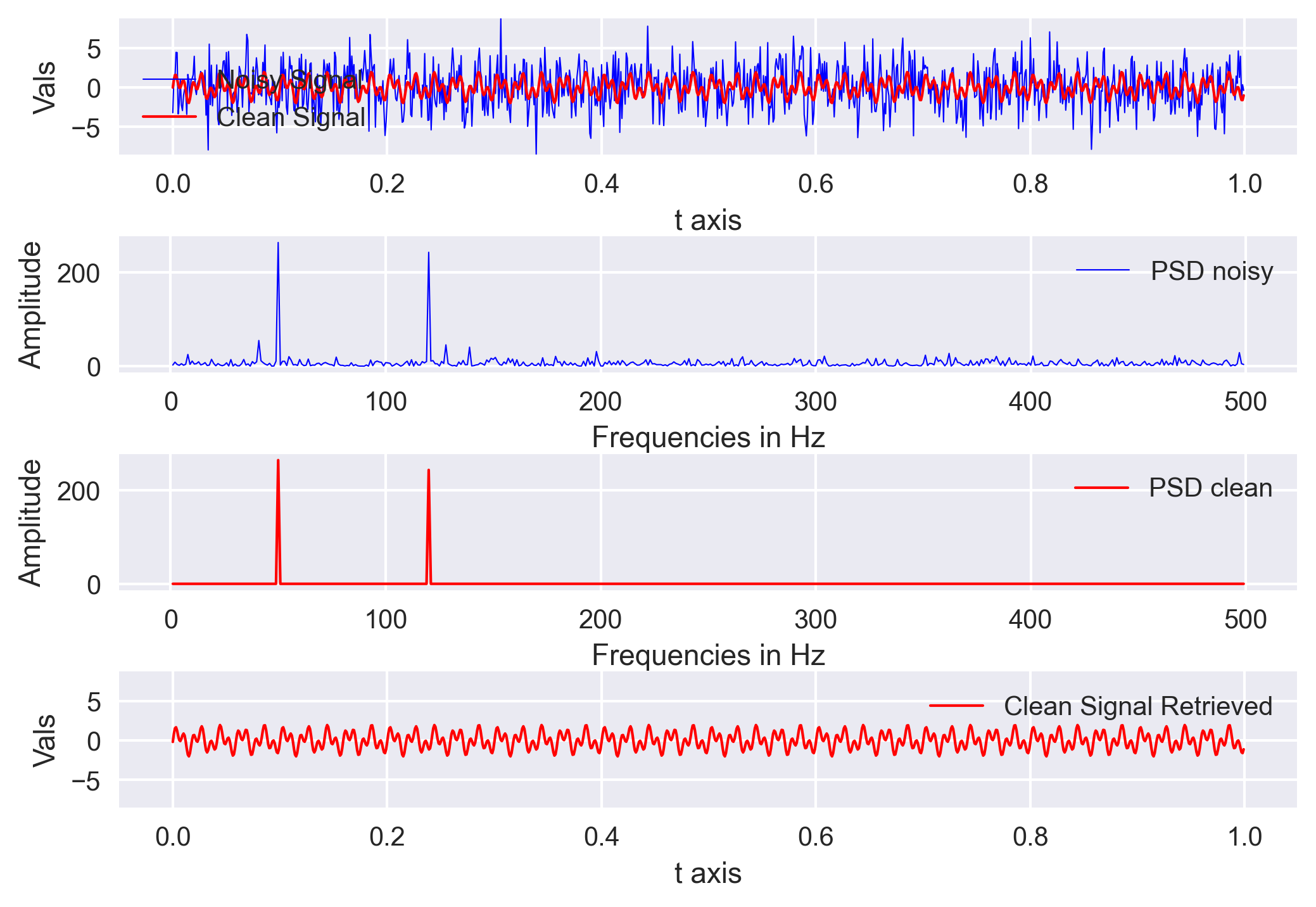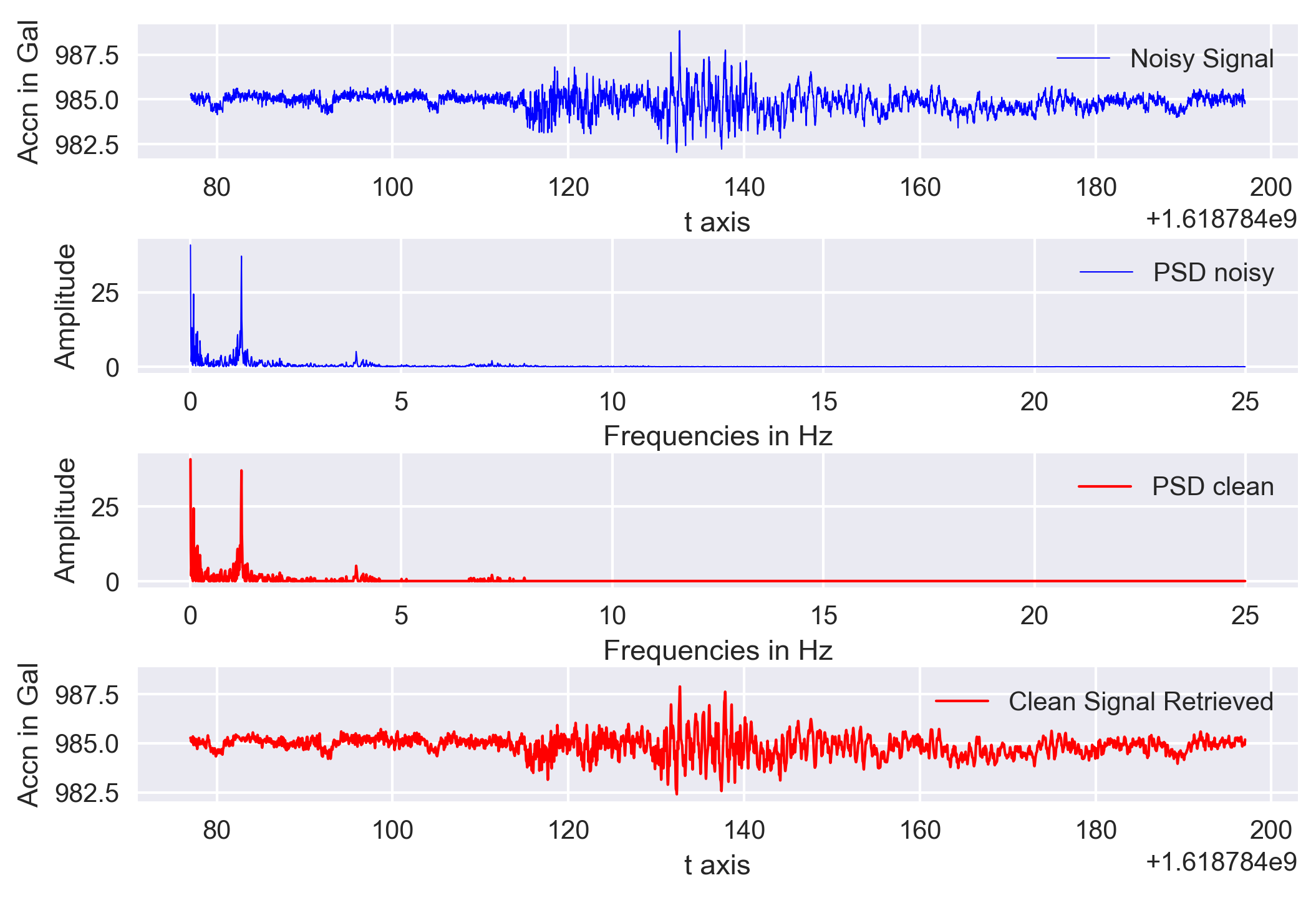# Signal denoising using Fourier Analysis in Python (codes included)

Utpal Kumar   5 minute read

We will learn the basics of Fourier analysis and implement it to remove noise from the synthetic and real signals

Fourier analysis is based on the idea that any time series can be decomposed into a sum of integral of harmonic waves of different frequencies. Hence, theoretically, we can employ a number of harmonic waves to generate any signal.

The Fourier series for an arbitrary function of time $f(t)$ defined over the interval ((-T/2 < t < T/2 )) is

\begin{equation}

f(t) = a_0 + \sum_{n=1}^{\infty} a_n cos(\frac{2n\pi t}{T}) + \sum_{n=1}^{\infty} b_n sin(\frac{2n\pi t}{T})

\end{equation}

In the above equation, we can see that the $sin(\frac{2n\pi t}{T})$ and $cos(\frac{2n\pi t}{T})$ are periodic with period $T/n$ or frequency $n/T$. Here, the larger values of $n$ correspond to shorter periods, or higher frequencies.

In this post, we will use Fourier analysis to filter with the assumption that noise is overlapping the signals in the time domain but are not so overlapping in the frequency domain.

## Import libraries, create a signal, and add noise

import pandas as pd
import os, sys
import numpy as np
import matplotlib.pyplot as plt
plt.rcParams['figure.figsize'] = [10,6]
plt.rcParams.update({'font.size': 18})
plt.style.use('seaborn')

## Create synthetic signal
dt = 0.001
t = np.arange(0, 1, dt)
signal = np.sin(2*np.pi*50*t) + np.sin(2*np.pi*120*t) #composite signal
signal_clean = signal #copy for later comparison
signal = signal + 2.5 * np.random.randn(len(t))
minsignal, maxsignal = signal.min(), signal.max()


We created our signal by summing two sine functions different frequencies (50Hz and 120Hz). Then we created an array of random noise and stacked that noise onto the signal.

## Perform Fast Fourier Transform

## Compute Fourier Transform
n = len(t)
fhat = np.fft.fft(signal, n) #computes the fft
psd = fhat * np.conj(fhat)/n
freq = (1/(dt*n)) * np.arange(n) #frequency array
idxs_half = np.arange(1, np.floor(n/2), dtype=np.int32) #first half index


Numpy’s fft.fft function returns the one-dimensional discrete Fourier Transform with the efficient Fast Fourier Transform (FFT) algorithm. The output of the function is complex and we multiplied it with its conjugate to obtain the power spectrum of the noisy signal. We created the array of frequencies using the sampling interval (dt) and the number of samples (n).Fast Fourier Transform applied on the noisy synthetic data

## Filter out the noise

In the above plot, we can see that the two frequecies from our original signal is standing out. Now, we can create a filter that can remove all frequencies with amplitude less than our threshold.

## Filter out noise
threshold = 100
psd_idxs = psd > threshold #array of 0 and 1
psd_clean = psd * psd_idxs #zero out all the unnecessary powers
fhat_clean = psd_idxs * fhat #used to retrieve the signal

signal_filtered = np.fft.ifft(fhat_clean) #inverse fourier transform


## Visualization the results

## Visualization
fig, ax = plt.subplots(4,1)
ax.plot(t, signal, color='b', lw=0.5, label='Noisy Signal')
ax.plot(t, signal_clean, color='r', lw=1, label='Clean Signal')
ax.set_ylim([minsignal, maxsignal])
ax.set_xlabel('t axis')
ax.set_ylabel('Vals')
ax.legend()

ax.plot(freq[idxs_half], np.abs(psd[idxs_half]), color='b', lw=0.5, label='PSD noisy')
ax.set_xlabel('Frequencies in Hz')
ax.set_ylabel('Amplitude')
ax.legend()

ax.plot(freq[idxs_half], np.abs(psd_clean[idxs_half]), color='r', lw=1, label='PSD clean')
ax.set_xlabel('Frequencies in Hz')
ax.set_ylabel('Amplitude')
ax.legend()

ax.plot(t, signal_filtered, color='r', lw=1, label='Clean Signal Retrieved')
ax.set_ylim([minsignal, maxsignal])
ax.set_xlabel('t axis')
ax.set_ylabel('Vals')
ax.legend()

plt.savefig('signal-analysis.png', bbox_inches='tight', dpi=300)Fast Fourier Transform applied on the noisy synthetic data

## Real data denoising using power threshold

I have a recording of the accelerometer data using the PhidgetSpatial Precision 0/0/3 High Resolution. I converted that into Miniseed format for easy analysis.

# -*- coding: utf-8 -*-
# ======================================================================================================================================================
"""
Created on Thu Apr 29 12:41:26 2021

@author: Utpal Kumar (IES, Academia Sinica)
"""
# ======================================================================================================================================================

import numpy as np
import matplotlib.pyplot as plt
plt.rcParams['figure.figsize'] = [10,6]
plt.rcParams.update({'font.size': 18})
plt.style.use('seaborn')
from obspy import read
from obspy.core import UTCDateTime

otime = UTCDateTime('2021-04-18T22:14:37') #eq origin

filenameZ = 'TW-RCEC7A-BNZ.mseed'

streams = [stZ.copy()]
traces = []
for st in streams:
tr = st.trim(otime, otime+120)
traces.append(tr)

delta = stZ.stats.delta
starttime = np.datetime64(stZ.stats.starttime)
endtime = np.datetime64(stZ.stats.endtime)
signalZ = traces.data/10**6
minsignal, maxsignal = signalZ.min(), signalZ.max()

t = traces.times("utcdatetime")

## Compute Fourier Transform
n = len(t)
fhat = np.fft.fft(signalZ, n) #computes the fft
psd = fhat * np.conj(fhat)/n
freq = (1/(delta*n)) * np.arange(n) #frequency array
idxs_half = np.arange(1, np.floor(n/2), dtype=np.int32) #first half index
psd_real = np.abs(psd[idxs_half]) #amplitude for first half

## Filter out noise
sort_psd = np.sort(psd_real)[::-1]
# print(len(sort_psd))
threshold = sort_psd
psd_idxs = psd > threshold #array of 0 and 1
psd_clean = psd * psd_idxs #zero out all the unnecessary powers
fhat_clean = psd_idxs * fhat #used to retrieve the signal

signal_filtered = np.fft.ifft(fhat_clean) #inverse fourier transform

## Visualization
fig, ax = plt.subplots(4,1)
ax.plot(t, signalZ, color='b', lw=0.5, label='Noisy Signal')
ax.set_xlabel('t axis')
ax.set_ylabel('Accn in Gal')
ax.legend()

ax.plot(freq[idxs_half], np.abs(psd[idxs_half]), color='b', lw=0.5, label='PSD noisy')
ax.set_xlabel('Frequencies in Hz')
ax.set_ylabel('Amplitude')
ax.legend()

ax.plot(freq[idxs_half], np.abs(psd_clean[idxs_half]), color='r', lw=1, label='PSD clean')
ax.set_xlabel('Frequencies in Hz')
ax.set_ylabel('Amplitude')
ax.legend()

ax.plot(t, signal_filtered, color='r', lw=1, label='Clean Signal Retrieved')
ax.set_ylim([minsignal, maxsignal])
ax.set_xlabel('t axis')
ax.set_ylabel('Accn in Gal')
ax.legend()

plt.savefig('real-signal-analysis.png', bbox_inches='tight', dpi=300)Fast Fourier Transform applied on the real data

## Obspy based filter

Obspy made our task much easier by introducing the filter functions. Here, I made use of the Butterworth-Bandpass filter. For details about different kinds of filters, you can see its documentation.

In this example, I used pass band low corner frequency of 0.01 and high corner frequency of 3 Hz based on the frequency spectrum obtained above.

import pandas as pd
import os, sys
import numpy as np
import matplotlib.pyplot as plt
plt.rcParams['figure.figsize'] = [10,6]
plt.rcParams.update({'font.size': 18})
plt.style.use('seaborn')
from obspy import read
from obspy.core import UTCDateTime

otime = UTCDateTime('2021-04-18T22:14:37') #eq origin

filenameZ = 'TW-RCEC7A-BNZ.mseed'

streams = [stZ.copy()]
traces = []
for st in streams:
tr = st.trim(otime, otime+120)
traces.append(tr)

signalZ = traces.data/10**6
minsignal, maxsignal = signalZ.min(), signalZ.max()

t = np.arange(0, traces.stats.npts / traces.stats.sampling_rate, traces.stats.delta)

# Filtering with a lowpass on a copy of the original Trace
freqmin = 0.01
freqmax = 3
tr_filt = traces.copy()
tr_filt.detrend("linear")
tr_filt.taper(max_percentage=0.05, type='hann')
tr_filt.filter("bandpass", freqmin=freqmin,
freqmax=freqmax, corners=4, zerophase=True)
print(tr_filt.data/10**6)
signal_filtered = tr_filt.data/10**6

## Visualization
fig, ax = plt.subplots(2,1)
ax.plot(t, signalZ, color='b', lw=0.5, label='Noisy Signal')
ax.set_xlabel('t axis')
ax.set_ylabel('Accn in Gal')
ax.legend()

ax.plot(t, signal_filtered, color='r', lw=1, label='Clean Signal Retrieved')
ax.set_xlabel('t axis')
ax.set_ylabel('Accn in Gal')
ax.set_title(f"Filtered in range {freqmin}-{freqmax} Hz")
ax.legend()